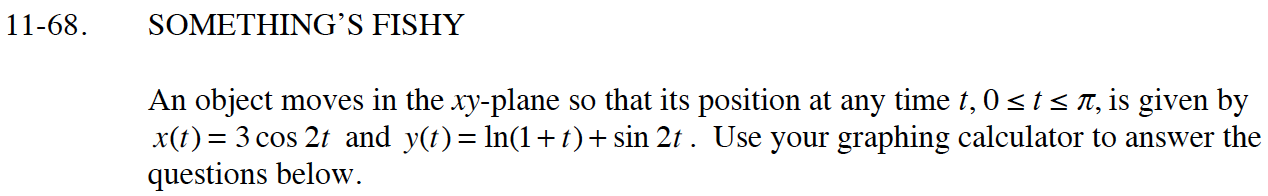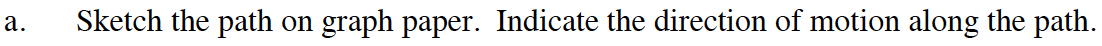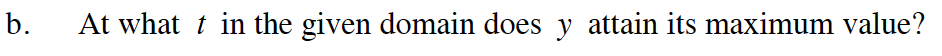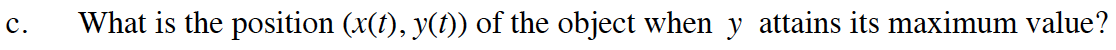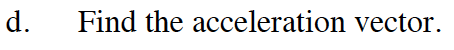### Home > CALC > Chapter 11 > Lesson 11.2.3 > Problem11-68

11-68.
1. SOMETHING'S FISHY
An object moves in the xy-plane so that its position at any time t, 0 ≤ tπ, is given by x(t) = 3 cos 2t and y(t) = ln(l + t) + sin 2t. Use your graphing calculator to answer the questions below. Homework Help ✎

1. Sketch the path on graph paper. Indicate the direction of motion along the path.

2. At what t in the given domain does y attain its maximum value?

3. What is the position (x(t), y(t)) of the object when y attains its maximum value?

4. Find the acceleration vector.